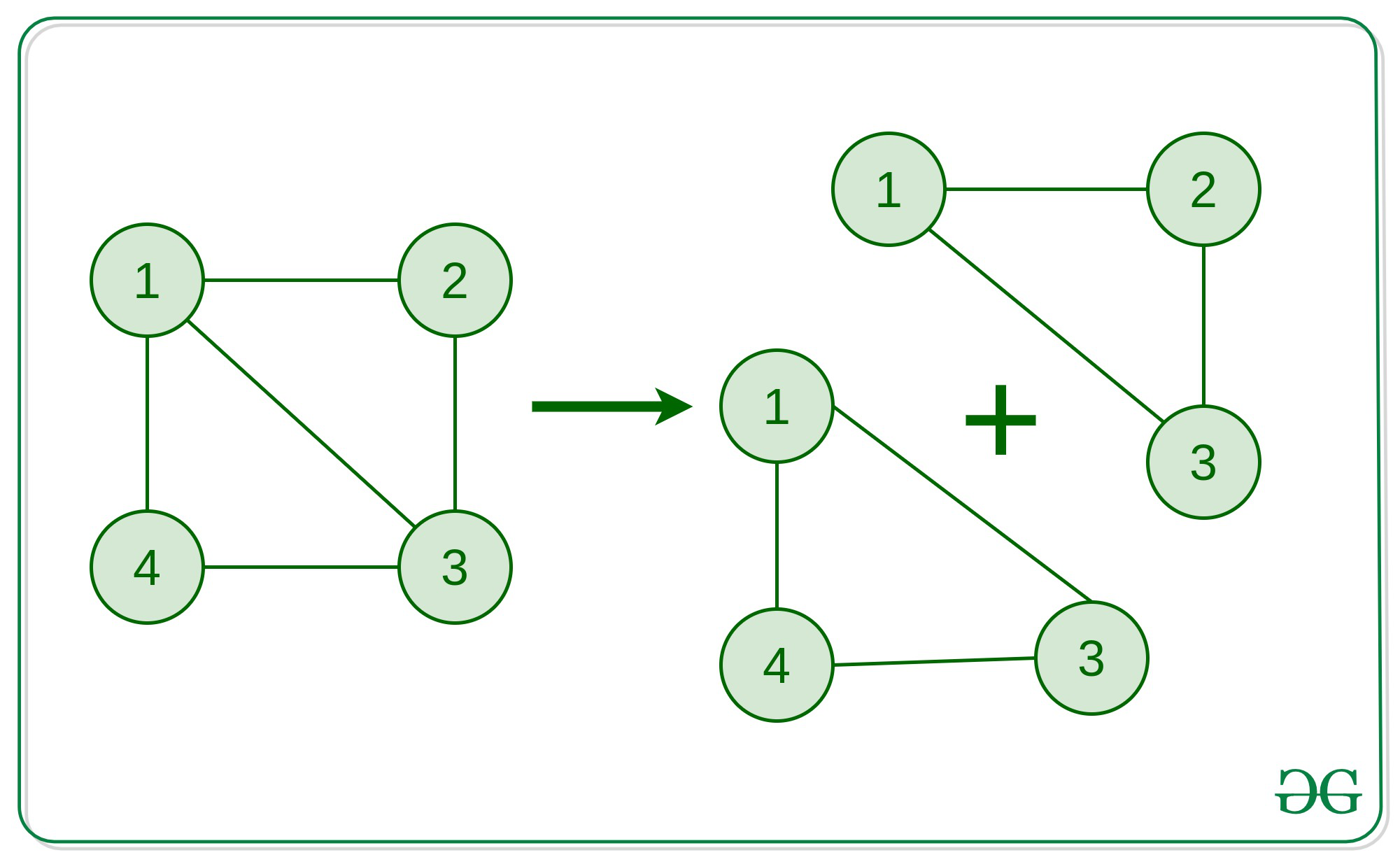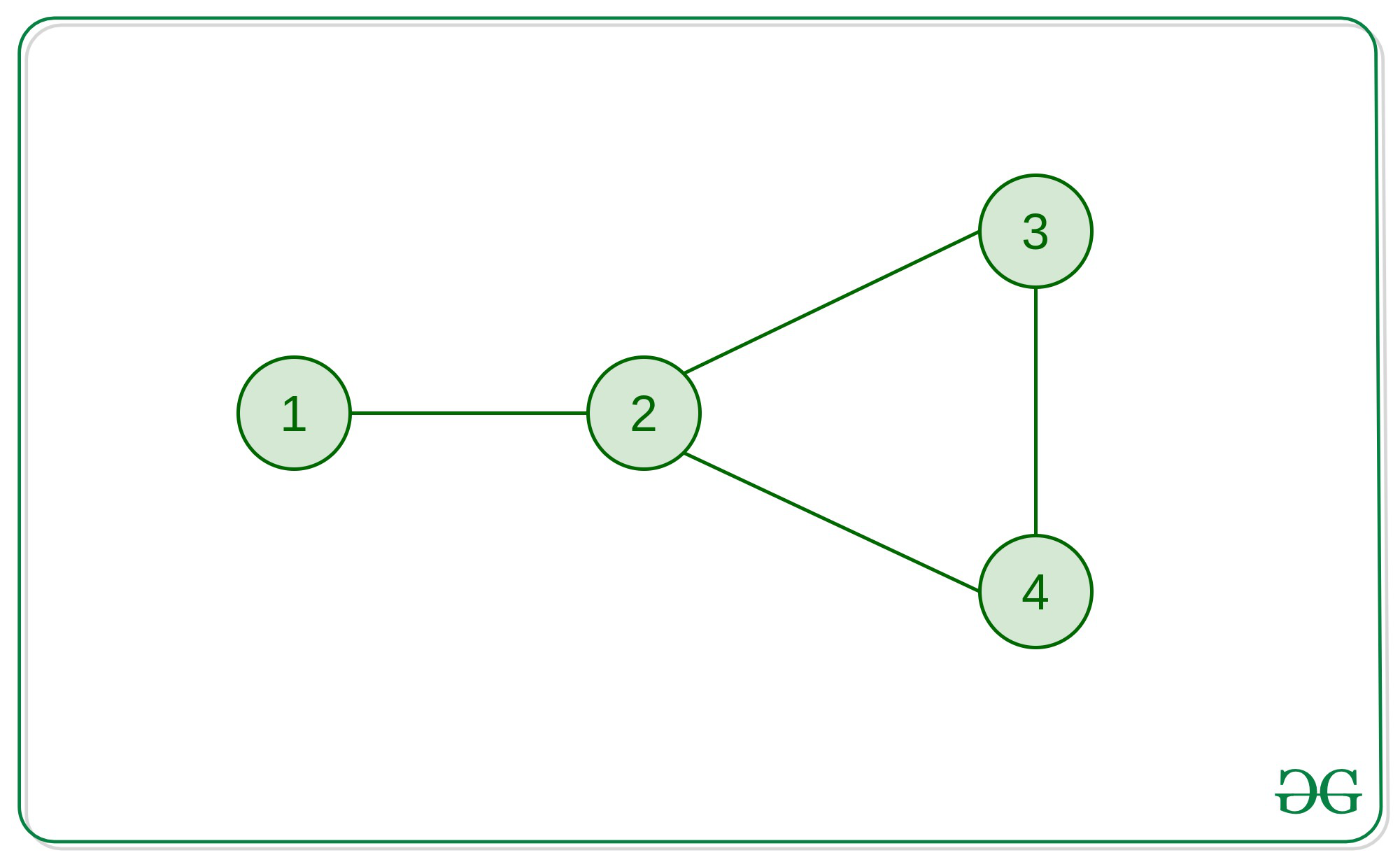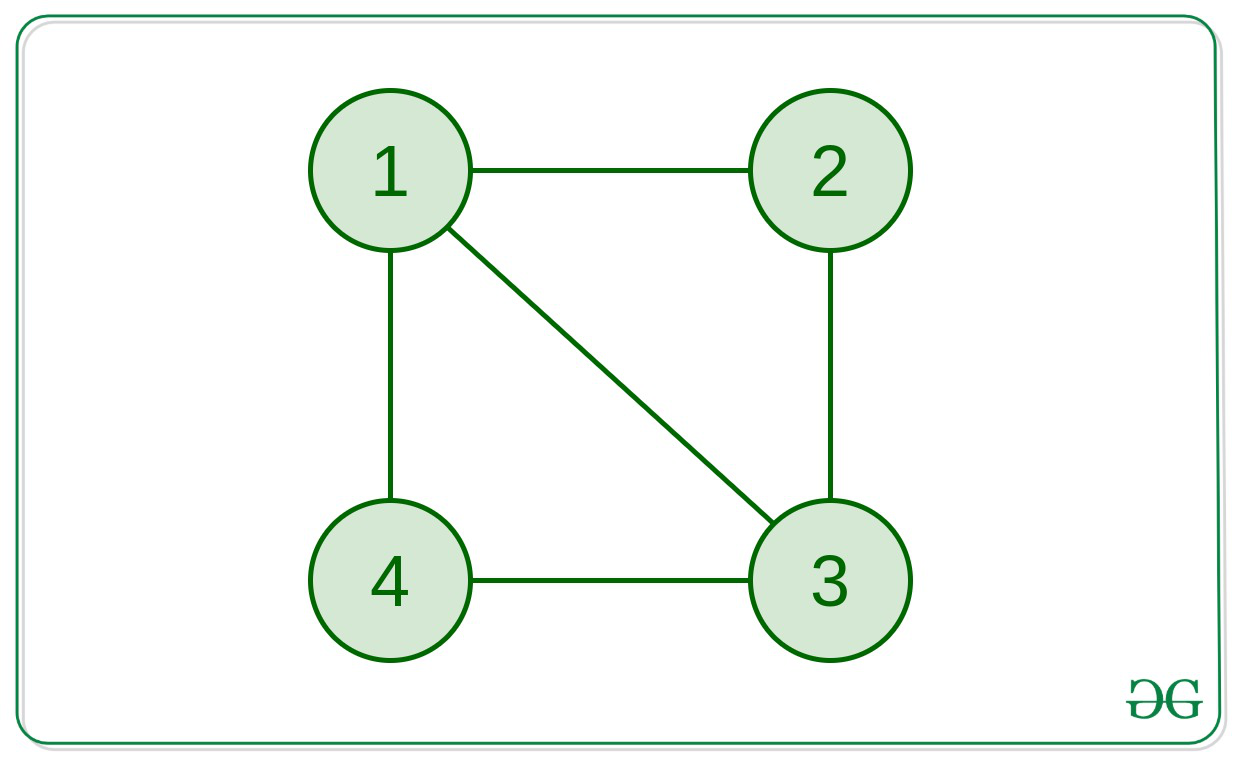Open in App
Not now

# Find any simple cycle in an undirected unweighted Graph

• Difficulty Level : Hard
• Last Updated : 06 Jan, 2023

Given an un-directed and unweighted connected graph, find a simple cycle in that graph (if it exists).

Simple Cycle:

A simple cycle is a cycle in a Graph with no repeated vertices (except for the beginning and ending vertex).

Basically, if a cycle can’t be broken down to two or more cycles, then it is a simple cycle.
For better understanding, refer to the following image:The graph in the above picture explains how the cycle 1 -> 2 -> 3 -> 4 -> 1 isn’t a simple cycle
because, it can be broken into 2 simple cycles 1 -> 3 -> 4 -> 1 and 1 -> 2 -> 3 -> 1.

Examples:

Input: edges[]  = {(1, 2), (2, 3), (2, 4), (3, 4)}Output: 2 => 3 => 4 => 2
Explanation:
This graph has only one cycle of length 3 which is a simple cycle.

Input: edges[] = {(1, 2), (2, 3), (3, 4), (1, 4), (1, 3)}Output: 1 => 3 => 4 => 1

Approach:  The idea is to check that if the graph contains a cycle or not. This can be done by simply using a DFS
Now, if the graph contains a cycle, we can get the end vertices (say a and b) of that cycle from the DFS itself. Now, if we run a BFS from a to b (ignoring the direct edge between a and b), we’ll be able to get the shortest path from a to b, which will give us the path of the shortest cycle containing the points a and b. The path can be easily tracked by using a parent array. This shortest cycle will be a simple cycle

#### Proof that the shortest cycle will be a simple cycle:

We can prove this using contradiction. Let’s say there exists another simple cycle inside this cycle. This means the inner simple cycle will have a shorter length and, hence it can be said that there’s a shorter path from a to b. But we have found the shortest path from a to b using BFS. Hence, no shorter path exists and the found path is the shortest. So, no inner cycles can exist inside of the cycle we’ve found.
Hence, this cycle is a simple cycle.

Below is the implementation of the above approach:

## C++

 `// C++ implementation to find the``// simple cycle in the given path` `#include ``using` `namespace` `std;``#define MAXN 1005` `// Declaration of the Graph``vector > adj(MAXN);` `// Declaration of visited array``vector<``bool``> vis(MAXN);``int` `a, b;` `// Function to add edges``// connecting 'a' and 'b'``// to the graph``void` `addedge(``int` `a, ``int` `b)``{``    ``adj[a].push_back(b);``    ``adj[b].push_back(a);``}` `// Function to detect if the``// graph contains a cycle or not``bool` `detect_cycle(``int` `node, ``int` `par)``{``    ``// Marking the current node visited``    ``vis[node] = 1;``    ``// Traversing to the childs``    ``// of the current node``    ``// Simple DFS approach``    ``for` `(``auto` `child : adj[node]) {``        ``if` `(vis[child] == 0) {``            ``if` `(detect_cycle(child, node))``                ``return` `true``;``        ``}` `        ``// Checking for a back-edge``        ``else` `if` `(child != par) {``            ``// A cycle is detected``            ``// Marking the end-vertices``            ``// of the cycle``            ``a = child;``            ``b = node;``            ``return` `true``;``        ``}``    ``}``    ``return` `false``;``}` `vector<``int``> simple_cycle;` `// Function to get the simple cycle from the``// end-vertices of the cycle we found from DFS``void` `find_simple_cycle(``int` `a, ``int` `b)``{``    ``// Parent array to get the path``    ``vector<``int``> par(MAXN, -1);` `    ``// Queue for BFS``    ``queue<``int``> q;``    ``q.push(a);``    ``bool` `ok = ``true``;``    ``while` `(!q.empty()) {``        ``int` `node = q.front();``        ``q.pop();``        ``vis[node] = 1;``        ``for` `(``auto` `child : adj[node]) {``            ``if` `(node == a && child == b)``                ``// Ignoring the direct edge``                ``// between a and b``                ``continue``;` `            ``if` `(vis[child] == 0) {``                ``// Updating the parent array``                ``par[child] = node;` `                ``if` `(child == b) {``                    ``// If b is reached,``                    ``// we've found the``                    ``// shortest path from``                    ``// a to b already``                    ``ok = ``false``;``                    ``break``;``                ``}``                ``q.push(child);``                ``vis[child] = 1;``            ``}``        ``}``        ``// If required task is done``        ``if` `(ok == ``false``)``            ``break``;``    ``}` `    ``// Cycle starting from a``    ``simple_cycle.push_back(a);``    ``int` `x = b;` `    ``// Until we reach a again``    ``while` `(x != a) {``        ``simple_cycle.push_back(x);``        ``x = par[x];``    ``}``}` `// Driver Code``int` `main()``{` `    ``// Creating the graph``    ``addedge(1, 2);``    ``addedge(2, 3);``    ``addedge(3, 4);``    ``addedge(4, 1);``    ``addedge(1, 3);` `    ``if` `(detect_cycle(1, -1) == ``true``) {``        ``// If cycle is present` `        ``// Resetting the visited array``        ``// for simple cycle finding``        ``vis = vector<``bool``>(MAXN, ``false``);``        ``find_simple_cycle(a, b);` `        ``// Printing the simple cycle``        ``cout << ``"A simple cycle: "``;``        ``for` `(``auto``& node : simple_cycle) {``            ``cout << node << ``" => "``;``        ``}``        ``cout << a;``        ``cout << ``"\n"``;``    ``}``    ``else` `{``        ``cout << ``"The Graph doesn't "``             ``<< ``"contain a cycle.\n"``;``    ``}` `    ``return` `0;``}`

## Java

 `// Java implementation to``// find the simple cycle``// in the given path``import` `java.util.*;``class` `GFG{` `  ``static` `final` `int` `MAXN = ``1005``;` `// Declaration of the``// Graph``static` `Vector []adj =``              ``new` `Vector[MAXN];` `// Declaration of visited``// array``static` `boolean` `[]vis =``       ``new` `boolean``[MAXN];``static` `int` `a, b;` `// Function to add edges``// connecting 'a' and 'b'``// to the graph``static` `void` `addedge(``int` `a,``                    ``int` `b)``{``  ``adj[a].add(b);``  ``adj[b].add(a);``}` `// Function to detect if the``// graph contains a cycle or not``static` `boolean` `detect_cycle(``int` `node,``                            ``int` `par)``{``  ``// Marking the current``  ``// node visited``  ``vis[node] = ``true``;``  ` `  ``// Traversing to the childs``  ``// of the current node``  ``// Simple DFS approach``  ``for` `(``int` `child : adj[node])``  ``{``    ``if` `(vis[child] == ``false``)``    ``{``      ``if` `(detect_cycle(child,``                       ``node))``        ``return` `true``;``    ``}` `    ``// Checking for a back-edge``    ``else` `if` `(child != par)``    ``{``      ``// A cycle is detected``      ``// Marking the end-vertices``      ``// of the cycle``      ``a = child;``      ``b = node;``      ``return` `true``;``    ``}``  ``}``  ``return` `false``;``}` `static` `Vector simple_cycle =``              ``new` `Vector<>();` `// Function to get the simple``// cycle from the end-vertices``//of the cycle we found from DFS``static` `void` `find_simple_cycle(``int` `a,``                              ``int` `b)``{``  ``// Parent array to get the path``  ``int` `[]par = ``new` `int``[MAXN];` `  ``// Queue for BFS``  ``Queue q =``        ``new` `LinkedList<>();``  ``q.add(a);``  ``boolean` `ok = ``true``;``  ` `  ``while` `(!q.isEmpty())``  ``{``    ``int` `node = q.peek();``    ``q.remove();``    ``vis[node] = ``true``;``    ` `    ``for` `(``int` `child : adj[node])``    ``{``      ``if` `(node == a &&``          ``child == b)``        ``// Ignoring the direct edge``        ``// between a and b``        ``continue``;` `      ``if` `(vis[child] == ``false``)``      ``{``        ``// Updating the parent``        ``// array``        ``par[child] = node;` `        ``if` `(child == b)``        ``{``          ``// If b is reached,``          ``// we've found the``          ``// shortest path from``          ``// a to b already``          ``ok = ``false``;``          ``break``;``        ``}``        ``q.add(child);``        ``vis[child] = ``true``;``      ``}``    ``}``    ``// If required task``    ``// is done``    ``if` `(ok == ``false``)``      ``break``;``  ``}` `  ``// Cycle starting from a``  ``simple_cycle.add(a);``  ``int` `x = b;` `  ``// Until we reach``  ``// a again``  ``while` `(x != a)``  ``{``    ``simple_cycle.add(x);``    ``x = par[x];``  ``}``}` `// Driver Code``public` `static` `void` `main(String[] args)``{``  ``for` `(``int` `i = ``0``; i < adj.length; i++)``    ``adj[i] = ``new` `Vector();``  ` `  ``// Creating the graph``  ``addedge(``1``, ``2``);``  ``addedge(``2``, ``3``);``  ``addedge(``3``, ``4``);``  ``addedge(``4``, ``1``);``  ``addedge(``1``, ``3``);` `  ``if` `(detect_cycle(``1``, -``1``) == ``true``)``  ``{``    ``// If cycle is present``    ``// Resetting the visited array``    ``// for simple cycle finding``    ``Arrays.fill(vis, ``false``);``    ``find_simple_cycle(a, b);` `    ``// Printing the simple cycle``    ``System.out.print(``"A simple cycle: "``);``    ` `    ``for` `(``int` `node : simple_cycle)``    ``{``      ``System.out.print(node + ``" => "``);``    ``}``    ``System.out.print(a);``    ``System.out.print(``"\n"``);``  ``}``  ``else``  ``{``    ``System.out.print(``"The Graph doesn't "` `+``                     ``"contain a cycle.\n"``);``  ``}``}``}` `// This code is contributed by shikhasingrajput`

## Python3

 `# Python3 implementation to find the``# simple cycle in the given path` `MAXN ``=` `1005`` ` `# Declaration of the Graph``adj ``=` `[[] ``for` `i ``in` `range``(MAXN)]`` ` `# Declaration of visited array``vis ``=` `[``False` `for` `i ``in` `range``(MAXN)]``aa ``=` `0``bb ``=` `0`` ` `# Function to add edges``# connecting 'a' and 'b'``# to the graph``def` `addedge(a, b):` `    ``adj[a].append(b);``    ``adj[b].append(a);``     ` `# Function to detect if the``# graph contains a cycle or not``def` `detect_cycle(node, par):``    ``global` `aa, bb` `    ``# Marking the current node visited``    ``vis[node] ``=` `True``;` `    ``# Traversing to the childs``    ``# of the current node``    ``# Simple DFS approach``    ``for` `child ``in` `adj[node]:``        ` `        ``if` `(vis[child] ``=``=` `False``):``            ``if` `(detect_cycle(child, node)):``                ``return` `True``;`` ` `        ``# Checking for a back-edge``        ``elif` `(child !``=` `par):` `            ``# A cycle is detected``            ``# Marking the end-vertices``            ``# of the cycle``            ``aa ``=` `child;``            ``bb ``=` `node;``            ``return` `True``;``        ` `    ``return` `False``;`` ` `simple_cycle ``=` `[]`` ` `# Function to get the simple cycle from the``# end-vertices of the cycle we found from DFS``def` `find_simple_cycle(a, b):` `    ``# Parent array to get the path``    ``par ``=` `[``0` `for` `i ``in` `range``(MAXN)]`` ` `    ``# Queue for BFS``    ``q ``=` `[]``    ``q.append(a);``    ``ok ``=` `True``;``    ``while``(``len``(q) !``=` `0``):``        ` `        ``node ``=` `q[``0``];``        ``q.pop(``0``);``        ``vis[node] ``=` `True``;``        ` `        ``for` `child ``in` `adj[node]:``            ` `            ``if` `(node ``=``=` `a ``and` `child ``=``=` `b):``                ` `                ``# Ignoring the direct edge``                ``# between a and b``                ``continue``;`` ` `            ``if` `(vis[child] ``=``=` `False``):``                ` `                ``# Updating the parent array``                ``par[child] ``=` `node;`` ` `                ``if` `(child ``=``=` `b):``                    ` `                    ``# If b is reached,``                    ``# we've found the``                    ``# shortest path from``                    ``# a to b already``                    ``ok ``=` `False``;``                    ``break``;``            ` `                ``q.append(child);``                ``vis[child] ``=` `True``;` `        ``# If required task is done``        ``if` `(ok ``=``=` `False``):``            ``break``;`` ` `    ``# Cycle starting from a``    ``simple_cycle.append(a);``    ``x ``=` `b;`` ` `    ``# Until we reach a again``    ``while` `(x !``=` `a):``        ``simple_cycle.append(x);``        ``x ``=` `par[x];``     ` `# Driver Code``if` `__name__``=``=``'__main__'``:``    ` `    ``# Creating the graph``    ``addedge(``1``, ``2``);``    ``addedge(``2``, ``3``);``    ``addedge(``3``, ``4``);``    ``addedge(``4``, ``1``);``    ``addedge(``1``, ``3``);`` ` `    ``if` `(detect_cycle(``1``, ``-``1``) ``=``=` `True``):``        ``# If cycle is present`` ` `        ``# Resetting the visited array``        ``# for simple cycle finding``        ``for` `i ``in` `range``(MAXN):``            ``vis[i] ``=` `False``        ``find_simple_cycle(aa, bb);` `        ``# Printing the simple cycle``        ``print``(``"A simple cycle: "``, end ``=` `'')``        ``for` `node ``in` `simple_cycle:``            ``print``(node, end ``=` `" => "``)``        ``print``(aa)` `    ``else``:``        ``print``(``"The Graph doesn't contain a cycle."``)` `        ``# This code is contributed by rutvik_56`

## C#

 `// C# implementation to``// find the simple cycle``// in the given path``using` `System;``using` `System.Collections.Generic;` `class` `GFG{``  ` `static` `readonly` `int` `MAXN = 1005;``  ` `// Declaration of the``// Graph``static` `List<``int``> []adj = ``new` `List<``int``>[MAXN];``  ` `// Declaration of visited``// array``static` `bool` `[]vis = ``new` `bool``[MAXN];``  ` `static` `int` `a, b;` `// Function to add edges``// connecting 'a' and 'b'``// to the graph``static` `void` `addedge(``int` `a, ``int` `b)``{``  ``adj[a].Add(b);``  ``adj[b].Add(a);``}` `// Function to detect if the``// graph contains a cycle or not``static` `bool` `detect_cycle(``int` `node,``                         ``int` `par)``{``  ` `  ``// Marking the current``  ``// node visited``  ``vis[node] = ``true``;``  ` `  ``// Traversing to the childs``  ``// of the current node``  ``// Simple DFS approach``  ``foreach``(``int` `child ``in` `adj[node])``  ``{``    ``if` `(vis[child] == ``false``)``    ``{``      ``if` `(detect_cycle(child,``                       ``node))``        ``return` `true``;``    ``}` `    ``// Checking for a back-edge``    ``else` `if` `(child != par)``    ``{``      ` `      ``// A cycle is detected``      ``// Marking the end-vertices``      ``// of the cycle``      ``a = child;``      ``b = node;``      ``return` `true``;``    ``}``  ``}``  ``return` `false``;``}` `static` `List<``int``> simple_cycle = ``new` `List<``int``>();``  ` `// Function to get the simple``// cycle from the end-vertices``//of the cycle we found from DFS``static` `void` `find_simple_cycle(``int` `a,``                              ``int` `b)``{``  ` `  ``// Parent array to get the path``  ``int` `[]par = ``new` `int``[MAXN];` `  ``// Queue for BFS``  ``Queue<``int``> q = ``new` `Queue<``int``>();``  ` `  ``q.Enqueue(a);``  ``bool` `ok = ``true``;``  ` `  ``while` `(q.Count != 0)``  ``{``    ``int` `node = q.Peek();``    ``q.Dequeue();``    ``vis[node] = ``true``;``    ` `    ``foreach``(``int` `child ``in` `adj[node])``    ``{``      ``if` `(node == a &&``          ``child == b)``        ` `        ``// Ignoring the direct edge``        ``// between a and b``        ``continue``;` `      ``if` `(vis[child] == ``false``)``      ``{``        ` `        ``// Updating the parent``        ``// array``        ``par[child] = node;` `        ``if` `(child == b)``        ``{``          ` `          ``// If b is reached,``          ``// we've found the``          ``// shortest path from``          ``// a to b already``          ``ok = ``false``;``          ``break``;``        ``}``        ``q.Enqueue(child);``        ``vis[child] = ``true``;``      ``}``    ``}``    ` `    ``// If required task``    ``// is done``    ``if` `(ok == ``false``)``      ``break``;``  ``}` `  ``// Cycle starting from a``  ``simple_cycle.Add(a);``  ``int` `x = b;` `  ``// Until we reach``  ``// a again``  ``while` `(x != a)``  ``{``    ``simple_cycle.Add(x);``    ``x = par[x];``  ``}``}` `// Driver Code``public` `static` `void` `Main(String[] args)``{``  ``for``(``int` `i = 0; i < adj.Length; i++)``    ``adj[i] = ``new` `List<``int``>();``  ` `  ``// Creating the graph``  ``addedge(1, 2);``  ``addedge(2, 3);``  ``addedge(3, 4);``  ``addedge(4, 1);``  ``addedge(1, 3);` `  ``if` `(detect_cycle(1, -1) == ``true``)``  ``{``    ` `    ``// If cycle is present``    ``// Resetting the visited array``    ``// for simple cycle finding``    ``for``(``int` `i = 0; i < vis.Length; i++)``        ``vis[i] = ``false``;``    ` `    ``find_simple_cycle(a, b);` `    ``// Printing the simple cycle``    ``Console.Write(``"A simple cycle: "``);``    ` `    ``foreach``(``int` `node ``in` `simple_cycle)``    ``{``      ``Console.Write(node + ``" => "``);``    ``}``    ``Console.Write(a);``    ``Console.Write(``"\n"``);``  ``}``  ``else``  ``{``    ``Console.Write(``"The Graph doesn't "` `+``                  ``"contain a cycle.\n"``);``  ``}``}``}` `// This code is contributed by gauravrajput1`

## Javascript

 ``

Output:

`A simple cycle: 1 => 4 => 3 => 1`

Time Complexity: O(V), where V is the number of vertices since we are doing just one DFS and BFS sequentially.
Auxiliary Space: O(MAXN)

My Personal Notes arrow_drop_up